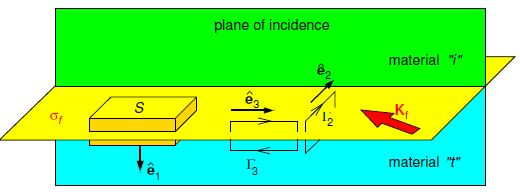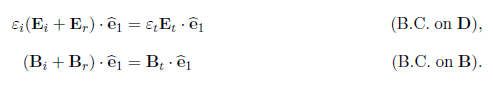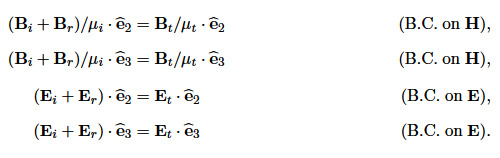Mathematics

# Explain Boundary Conditions at Interface

As an electromagnetic wave intersects the interface between two dielectrics, it is the boundary conditions on the electromagnetic field that determine the properties of the reflected and trans-mitted waves. In the dielectric where the incident wave is present, the electric and magnetic fields are the vector sums of those of the incident and reflected waves. The boundary conditions that need satisfying can be derived from Maxwell’s equations in integral form and are the same as were derived in “Essential Electromagnetism”. The relevant geometry is shown in below Figure together with the Gaussian pillbox and Amperian loops to be used.Figure: Gaussian pillbox and Amperian loops straddling the interface to be used in determining the boundary conditions on the EM wave fields.

The Gaussian pillbox is to be used with Gauss’ law ʃ D • dS = qf, encl and the no-magnetic-charge law ʃ B • dS = 0. The pillbox is infinitesimally thin and so we are only concerned with free surface charge, but in our case, we have σf = 0. Hence,The Amperian loops are to be used with Ampere’s law (as modified) ʃ H * dr = ʃ (Jf  + δD/δt) • dS and Faraday’s law ʃ E • dr = — ʃ δD/δt • dS. The Amperian loops are infinitesimally thin such that the contributions of δD/δt and δB/δt are negligible, and so we are only concerned with free surface currents but in our case Kf = 0, so,For the equations involving the magnetic field, we can substitute B = (n/c)E. Thus, for a monochromatic plane EM wave in a non-magnetic material, for which µ ≈ μ0 such that Ԑ ≈ n2 Ԑ0, we have a set of six simultaneous equations for Er and Et to be solved in terms of the (known) amplitude the electric field of the incident wave Ei, its angle of incidence O and the refractive indices of the two dielectrics. For the cases of polarisation perpendicular to, or parallel to, the plane of incidence, three of the six equations are identity equations leaving three equations (one of these three being redundant) which we will solve in the following sections.